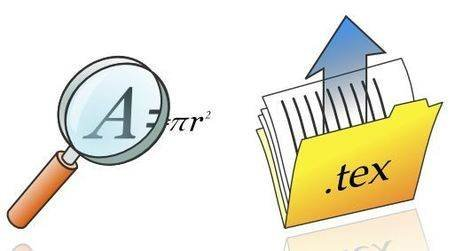# How to Search, Share and Manage Formulas with formulasheet.com

formulasheet.com is a web application which helps us in searching, sharing, and managing different formulas online. It is very useful for students, Professors or for those who are preparing for competing exams. This is very easy and exciting application. If you are able to create a new formula from all the formulas present to you, it also lets you to create the formula from scraps using its equation editor.

How does formulasheet.com help us:

It helps you in many ways. Some of them are mentioned below.

• Search Wikipedia or formulasheet for formulas.
• upload formulas as Latex file or copy the formulas to Latex.
• Combining the existing formulas with images or texts and arranging them in lists.How to get formulasheet.com app:

you can simply visit to its official site on http://formulasheet.com and then create an account there for free. Once you have an account at formulasheet.com, then browse, search, share and manage your formulas. This application is very useful if you use different formulas at work.

How does some of the best features of this application work are described below:

It is basically a collection of different sections. In each section you will find exciting thing about itself.

• Sheet:  This section of formulasheet.com shows the work you have done for yourself i.e. it simply open the page of formulas created or stored by you in your account. You can modify your existing sheet and can add more formulas or even pictures. If you wish you can take a print-out of this sheet
• My stuff:  This section helps in managing your formulas at some fixed place in some sheets or as lists as explained above in sheet. You can also share your formula to the people of outside world. Along-with it you can delete, render or copy formulas here to My stuff.
• Results:   This is the most important section of this app as this contain all the required formulas in itself which are necessary for you. It is a collection of all basic formulas from Mathematics, Physics and some other subjects as well as some of the advanced formulas are also there.  As this application doesn’t have its own database, it uses Wikipedia to get the different formulas to you. There is also an exciting feature of this and that is it gives you a typing suggestion while you were typing some of the formulas.
• Browse: This is a kind of tab in the formulasheet displays different engineering discipline subjects like : physics, Chemical engineering, Mathematics, Economics etc. It helps us a lot in saving time if we were willing to know about a specific discipline’s formula.

Thus, Formulasheet.com is overall a nice thing where we could simply store our formulas and whenever it is required we can see them at this app. This is a must app to try for those who are students as well as for those who uses formula at work.

Topic:  Online Tools